JMSLTM Numerical Library 7.2.0
com.imsl.math

## Class CsAkima

• All Implemented Interfaces:
Serializable, Cloneable

```public class CsAkima
extends Spline```
Extension of the Spline class to handle the Akima cubic spline.

Class `CsAkima` computes a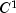cubic spline interpolant to a set of data points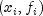for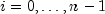. The breakpoints of the spline are the abscissas. Endpoint conditions are automatically determined by the program; see Akima (1970) or de Boor (1978).

If the data points arise from the values of a smooth, say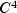, function f, i.e.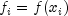, then the error will behave in a predictable fashion. Letbe the breakpoint vector for the above spline interpolant. Then, the maximum absolute error satisfies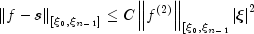where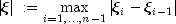`CsAkima` is based on a method by Akima (1970) to combat wiggles in the interpolant. The method is nonlinear; and although the interpolant is a piecewise cubic, cubic polynomials are not reproduced. (However, linear polynomials are reproduced.)

See Also:
Example, Serialized Form

• ### Fields inherited from class com.imsl.math.Spline

`breakPoint, coef, EPSILON_LARGE`
• ### Constructor Summary

Constructors
Constructor and Description
```CsAkima(double[] xData, double[] yData)```
Constructs the Akima cubic spline interpolant to the given data points.

• ### Methods inherited from class com.imsl.math.Spline

`copyAndSortData, copyAndSortData, derivative, derivative, derivative, getBreakpoints, integral, value, value`
• ### Methods inherited from class java.lang.Object

`clone, equals, finalize, getClass, hashCode, notify, notifyAll, toString, wait, wait, wait`
• ### Constructor Detail

• #### CsAkima

```public CsAkima(double[] xData,
double[] yData)```
Constructs the Akima cubic spline interpolant to the given data points.
Parameters:
`xData` - a `double` array containing the x-coordinates of the data. Values must be distinct.
`yData` - a `double` array containing the y-coordinates of the data.
Throws:
`IllegalArgumentException` - This exception is thrown if the arrays xData and yData do not have the same length.
JMSLTM Numerical Library 7.2.0

Copyright © 1970-2015 Rogue Wave Software
Built October 13 2015.